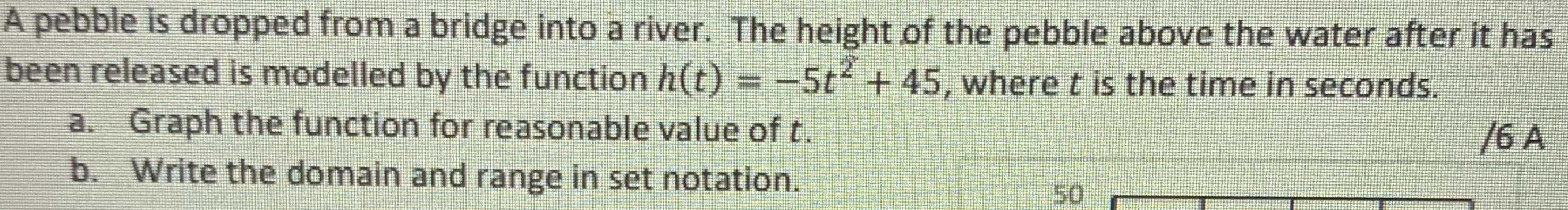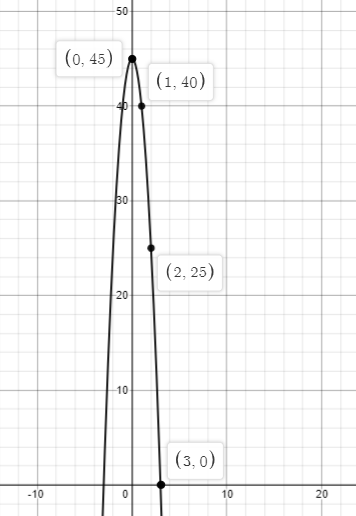### ¿Todavía tienes preguntas de matemáticas?

Pregunte a nuestros tutores expertos
Algebra
PreguntaA pebble is dropped from a bridge into a river. The height of the pebble above the water after it has been released is modelled by the function $$h ( t ) = - 5 t ^ { 2 } + 45$$ , where $$t$$ is the time in seconds. a. Graph the function for reasonable value of $$t$$ . b. Write the domain and range in set notation.